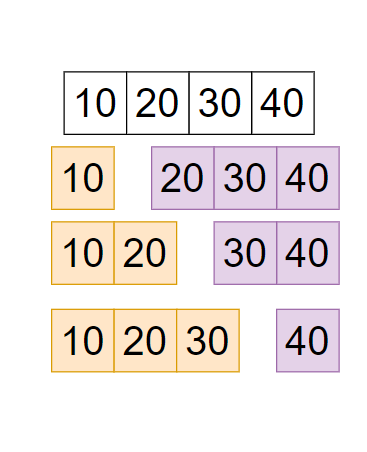15

# Allocate Books

Difficulty: MEDIUM
Avg. time to solve
10 min
Success Rate
90%

Problem Statement
Suggest Edit

#### ‘arr = { 10, 20, 30, 40 }’.#### But you have to return a minimum of this so return ‘min(90,70,60) =60’.

##### Note:
``````1. Do not print anything, just return the maximum number of pages that are assigned to a student is minimum.
2. If it is not possible to assign the ‘n’ books to ‘m’ students then return ‘-1’.
``````
##### Input format:
``````The first line of input contains an integer ‘T’ denoting the number of test cases.
The next ‘2*T’ lines represent the ‘T’ test cases.

The first line of each test case contains two space-separated integers ‘n’ denoting the number of books and ‘m’ denotes the number of students.

The second line of each test case contains ‘n’ space-separated integers denoting the number of pages in each of ‘n’ books.
``````
##### Output Format
``````For each test case, return the minimum number of pages.
``````
##### Constraints:
``````1 <= T <= 50
2 <= M <= N <= 10^3
1 <= A[i] <= 10^9
Sum of all A[i] do not more than 10^9.

Where ‘T’ is the total number of test cases, ‘N’ denotes the number of books and ‘M’ denotes the number of students. ‘A[i]’ denotes an element at position ‘i’ in the sequence.

Time limit: 1 second
``````
##### Sample Input 1:
``````2
4 2
12 34 67 90
4 4
5 17 100 11
``````
##### Sample Output 1:
``````113
100
``````
##### Explanation of sample input 1:
``````Test Case 1:

Let’s consider ‘n=4’ (number of books ) and ‘m=2’ (number of students)
‘arr = { 12, 34, 67, 90 }’. And ‘m= 2’.
All possible way to allocate the ‘4’ books in ‘2’ number of students is-

12 | 34, 67, 90 - sum of all the pages of books which allocated to student 1 is ‘12’, and student two is ‘34+ 67+ 90 = 191’ so maximum is ‘max(12, 191)= 191’.
12, 34 | 67, 90 - sum of all the pages of books which allocated to student 1 is ‘12+ 34 = 46’, and student two is ‘67+ 90 = 157’ so maximum is ‘max(46, 157)= 157’.
12, 34, 67 | 90 - sum of all the pages of books which allocated to student 1 is ‘12+ 34 +67 = 113’, and student two is ‘90’ so maximum is ‘max(113, 90)= 113’.

So possible maximum number of pages which allocated to a single student is { 191, 157, 113 }
But you have to return a minimum of this so return ‘min(191,157, 113) =113’.

Test Case 2:

‘arr = { 5, 17, 100, 11 }’. And ‘m=4’.
Only one is possible to allocate the ‘4’ books in ‘4’ student is

5 | 17 | 100 | 11 - maximum is ‘max(5, 17, 100, 11)= 100’.

``````
##### Sample Input 2:
``````2
5 4
25 46 28 49 24
3 2
10 10 20
``````
##### Sample Output 2:
``````71
20
``````Console